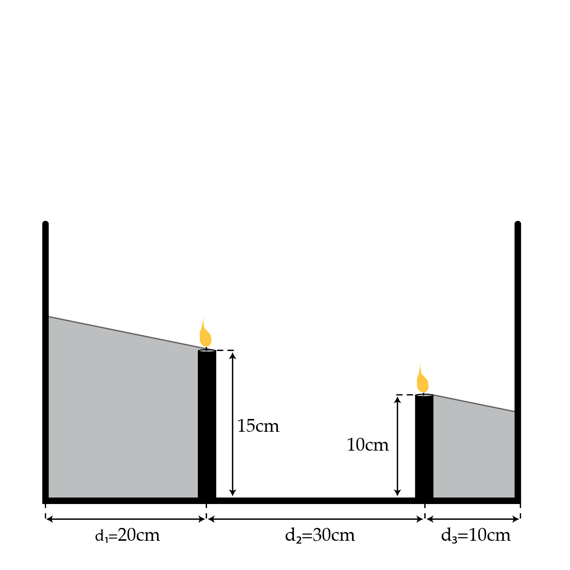# A romantic evening of physics

Calculus Level 4

Two candles are placed on a table between two parallel walls that are perpendicular to the ground. The line that passes through the bottom of these candles (which are on the ground) is also perpendicular to the walls.

These two candles separate the space between the walls into three regions of length $d_1=20~\mbox{cm}$, $d_2=30~\mbox{cm}$, $d_3=10~\mbox{cm}$ (from the left to right).

The candle on the left is Candle 1, and the other is Candle 2. The height of Candle 1 is $h_1=15~\mbox{cm}$ and Candle 2 is $h_2=10~\mbox{cm}$.

Now light these candles - their height shrinks with time. The rate at which Candle 1's height shrinks is 2 times faster than Candle 2's rate.

The question:

On the left wall, we see the shadow of Candle 1; on the right wall we see the shadow of Candle 2. Find the ratio of the speed of the Candle 1 shadow's top to Candle 2 shadow's top.×